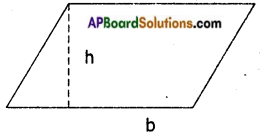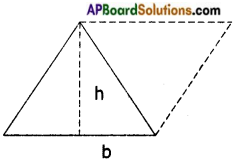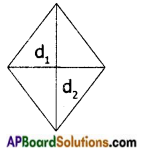# AP Board 7th Class Maths Notes Chapter 13 Area and Perimeter

Students can go through AP Board 7th Class Maths Notes Chapter 13 Area and Perimeter to understand and remember the concepts easily.

## AP State Board Syllabus 7th Class Maths Notes Chapter 13 Area and Perimeter

→ The area of a parallelogram is equal to the product of its base (b) and corresponding height (h) A = bh.Any side of the parallelogram can be taken as its base.

→ The area of a triangle is equal to half the product of its base and height.A = $$\frac{1}{2}$$ bh
A triangle = Half a parallelogram

→ The area of a Rhombus is equal to half the product of Its diagonals.A = $$\frac{1}{2}$$ d1d2

→ The circumference of a circle = 2πr = πd where π = $$\frac{22}{7}$$ or 3.14, d = $$\frac{r}{2}$$